The GLM Procedure
 PROC GLM Statement
PROC GLM <options> ;

The PROC GLM statement starts the GLM procedure. You can specify the following options in the PROC GLM statement.

ALPHA=p

specifies the level of significancefor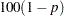% confidence intervals. The value must be between 0 and 1; the default value of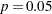results in 95% intervals. This value is used as the default confidence level for limits computed by the following options.

 Statement Options UCL= LCL= UCLM= LCLM=

You can override the default in each of these cases by specifying the ALPHA= option for each statement individually.

DATA=SAS-data-set

names the SAS data set used by the GLM procedure. By default, PROC GLM uses the most recently created SAS data set.

MANOVA

requests the multivariate mode of eliminating observations with missing values. If any of the dependent variables have missing values, the procedure eliminates that observation from the analysis. The MANOVA option is useful if you use PROC GLM in interactive mode and plan to perform a multivariate analysis.

MULTIPASS

requests that PROC GLM reread the input data set when necessary, instead of writing the necessary values of dependent variables to a utility file. This option decreases disk space usage at the expense of increased execution times, and is useful only in rare situations where disk space is at an absolute premium.

NAMELEN=specifies the length of effect names in tables and output data sets to becharacters long, whereis a value between 20 and 200 characters. The default length is 20 characters.

NOPRINT

suppresses the normal display of results. The NOPRINT option is useful when you want only to create one or more output data sets with the procedure. Note that this option temporarily disables the Output Delivery System (ODS); see Chapter 20, Using the Output Delivery System, for more information.

ORDER=DATA | FORMATTED | FREQ | INTERNAL

specifies the order in which to sort the levels of the classification variables (which are specified in the CLASS statement). This ordering determines which parameters in the model correspond to each level in the data, so the ORDER= option can be useful when you specify the CONTRAST or ESTIMATE statement.

This option applies to the levels for all classification variables, except when you use the (default) ORDER=FORMATTED option with numeric classification variables that have no explicit format. With this option, the levels of such variables are ordered by their internal value.

The ORDER= option can take the following values:

Value of ORDER=

Levels Sorted By

DATA

Order of appearance in the input data set

FORMATTED

External formatted value, except for numeric variables with no explicit format, which are sorted by their unformatted (internal) value

FREQ

Descending frequency count; levels with the most observations come first in the order

INTERNAL

Unformatted value

By default, ORDER=FORMATTED. For FORMATTED and INTERNAL, the sort order is machine-dependent.

For more information about sorting order, see the chapter on the SORT procedure in the Base SAS Procedures Guide and the discussion of BY-group processing in SAS Language Reference: Concepts.

OUTSTAT=SAS-data-set

names an output data set that contains sums of squares, degrees of freedom,statistics, and probability levels for each effect in the model, as well as for each CONTRAST that uses the overall residual or error mean square (MSE) as the denominator in constructing thestatistic. If you use the CANONICAL option in the MANOVA statement and do not use an M= specification in the MANOVA statement, the data set also contains results of the canonical analysis.

PLOTS <(global-plot-options)> <= plot-request <(options)>>
PLOTS <(global-plot-options)> <= (plot-request <(options)> <... plot-request <(options)>>)>

controls the plots produced through ODS Graphics. When you specify only one plot request, you can omit the parentheses from around the plot request. For example:

```   PLOTS=NONE
PLOTS=(DIAGNOSTICS RESIDUALS)
PLOTS(UNPACK)=RESIDUALS
PLOT=MEANPLOT(CLBAND)
```

You must enable ODS Graphics before requesting plots, as in the following statements.

```ods graphics on;
proc glm data=iron;
model loss=fe fe*fe;
run;
ods graphics off;
```

For general information about ODS Graphics, see Chapter 21, Statistical Graphics Using ODS. If you have enabled ODS Graphics but do not specify the PLOTS= option, then PROC GLM produces a default set of plots, which might be different for different models, as discussed in the following.

• If you specify a one-way analysis of variance model, with just one CLASS variable, the GLM procedure will produce a grouped box plot of the response values versus the CLASS levels. For an example of the box plot, see the section One-Way Layout with Means Comparisons.

• If you specify a two-way analysis of variance model, with just two CLASS variables, the GLM procedure will produce an interaction plot of the response values, with horizontal position representing one CLASS variable and marker style representing the other; and with predicted response values connected by lines representing the two-way analysis. For an example of the interaction plot, see the section PROC GLM for Unbalanced ANOVA.

• If you specify a model with a single continuous predictor, the GLM procedure will produce a fit plot of the response values versus the covariate values, with a curve representing the fitted relationship and a band representing the confidence limits for individual mean values. For an example of the fit plot, see the section PROC GLM for Quadratic Least Squares Regression.

• If you specify a model with two continuous predictors and no CLASS variables, the GLM procedure will produce a contour fit plot, overlaying a scatter plot of the data and a contour plot of the predicted surface.

• If you specify an analysis of covariance model, with one or two CLASS variables and one continuous variable, the GLM procedure will produce an analysis of covariance plot of the response values versus the covariate values, with lines representing the fitted relationship within each classification level. For an example of the analysis of covariance plot, see Example 39.4.

• If you specify an LSMEANS statement with the PDIFF option, the GLM procedure will produce a plot appropriate for the type of LS-means comparison. For PDIFF=ALL (which is the default if you specify only PDIFF), the procedure produces a diffogram, which displays all pairwise LS-means differences and their significance. The display is also known as a “mean-mean scatter plot” (Hsu 1996). For PDIFF=CONTROL, the procedure produces a display of each noncontrol LS-mean compared to the control LS-mean, with two-sided confidence intervals for the comparison. For PDIFF=CONTROLL and PDIFF=CONTROLU a similar display is produced, but with one-sided confidence intervals. Finally, for the PDIFF=ANOM option, the procedure produces an "analysis of means" plot, comparing each LS-mean to the average LS-mean.

• If you specify a MEANS statement, the GLM procedure will produce a grouped box plot of the response values versus the effect for which means are being calculated.

The global plot options include the following:

ONLY

suppresses the default plots. Only plots specifically requested are displayed.

UNPACKPANEL
UNPACK

suppresses paneling. By default, multiple plots can appear in some output panels. Specify UNPACKPANEL to get each plot in a separate panel. You can specify PLOTS(UNPACKPANEL) to just unpack the default plots. You can also specify UNPACKPANEL as a suboption with DIAGNOSTICS and RESIDUALS.

The following individual plots and plot options are available. If you specify only one plot, then you can omit the parentheses.

ALL

produces all appropriate plots. You can specify other options with ALL; for example, to request all plots and unpack just the residuals, specify: PLOTS=(ALL RESIDUALS(UNPACK)).

ANCOVAPLOT<(CLM CLI LIMITS)>

modifies the analysis of covariance plot produced by default when you have an analysis of covariance model, with one or two CLASS variables and one continuous variable. By default the plot does not show confidence limits around the predicted values. The PLOTS=ANCOVAPLOT(CLM) option adds limits for the expected predicted values, and PLOTS=ANCOVAPLOT(CLI) adds limits for new predictions. Use PLOTS=ANCOVAPLOT(LIMITS) to add both kinds of limits.

ANOMPLOT

requests an analysis of means display, in which least squares means are compared against an average least squares mean (Ott 1967; Nelson 1982, 1991, 1993). LS-mean ANOM plots are produced only if you also specify PDIFF=ANOM or ADJUST=NELSON in the LSMEANS statement, and in this case they are produced by default.

BOXPLOT<(NPANELPOS=n)>

modifies the plot produced by default for the model effect in a one-way analysis of variance model, or for an effect specified in the MEANS statement. Suppose the effect haslevels. By default, or if you specify PLOTS=BOXPLOT(NPANELPOS=0), alllevels of the effect are displayed in a single plot. Specifying a nonzero value ofwill result in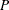panels, whereis the integer part of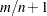. If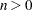, then the levels will be approximately balanced across thepanels; whereas if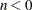, precisely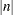levels will be displayed on each panel except possibly the last.

CONTOURFIT<(OBS=obs-options)>
modifies the contour fit plot produced by default when you have a model involving only two continuous predictors. The plot displays a contour plot of the predicted surface overlaid with a scatter plot of the observed data. You can use the following obs-options to control how the observations are displayed:

specifies that observations are displayed as circles colored by the observed response. The same color gradient is used to display the fitted surface and the observations. Observations where the predicted response is close to the observed response have similar colors: the greater the contrast between the color of an observation and the surface, the larger the residual is at that point.

OBS=NONE

suppresses the observations.

OBS=OUTLINE

specifies that observations are displayed as circles with a border but with a completely transparent fill.

is the same as OBS=GRADIENT except that a border is shown around each observation. This option is useful to identify the location of observations where the residuals are small, since at these points the color of the observations and the color of the surface are indistinguishable. OBS=OUTLINEGRADIENT is the default if you do not specify any obs-options.

CONTROLPLOT

requests a display in which least squares means are compared against a reference level. LS-mean control plots are produced only when you specify PDIFF=CONTROL or ADJUST=DUNNETT in the LSMEANS statement, and in this case they are produced by default.

DIAGNOSTICS<(LABEL UNPACK)>

requests that a panel of summary diagnostics for the fit be displayed. The panel displays scatter plots of residuals, absolute residuals, studentized residuals, and observed responses by predicted values; studentized residuals by leverage; Cook’s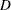by observation; a Q-Q plot of residuals; a residual histogram; and a residual-fit spread plot. The LABEL option displays labels on observations satisfying RSTUDENT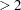, LEVERAGE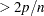, and on the Cook’splot, COOKSD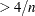, whereis the number of observations used in fitting the model, andis the number of parameters in the model. The label is the first ID variable if the ID statement is specified; otherwise, it is the observation number. The UNPACK option unpanels the diagnostic display and produces the series of individual plots that form the paneled display.

DIFFPLOT<(ABS NOABS CENTER NOLINES)>

modifies the plot produced by an LSMEANS statement with the PDIFF=ALL option (or just PDIFF, since ALL is the default argument). The ABS and NOABS options determine the positioning of the line segments in the plot. When the ABS option is in effect, and this is the default, all line segments are shown on the same side of the reference line. The NOABS option separates comparisons according to the sign of the difference. The CENTER option marks the center point for each comparison. This point corresponds to the intersection of two least squares means. The NOLINES option suppresses the display of the line segments that represent the confidence bounds for the differences of the least squares means. The NOLINES option implies the CENTER option. The default is to draw line segments in the upper portion of the plot area without marking the center point.

FITPLOT<(NOCLM NOCLI NOLIMITS)>

modifies the fit plot produced by default when you have a model with a single continuous predictor. By default the plot includes confidence limits for both the expected predicted values and individual new predictions. The PLOTS=FITPLOT(NOCLM) option removes the limits on the expected values and the PLOTS=FITPLOT(NOCLI) option removes the limits on new predictions. The PLOTS=FITPLOT(NOLIMITS) option removes both kinds of confidence limits.

INTPLOT<(CLM CLI LIMITS)>

modifies the interaction plot produced by default when you have a two-way analysis of variance model, with just two CLASS variables. By default the plot does not show confidence limits around the predicted values. The PLOTS=INTPLOT(CLM) option adds limits for the expected predicted values and PLOTS=INTPLOT(CLI) adds limits for new predictions. Use PLOTS=ANCOVAPLOT(LIMITS) to add both kinds of limits.

MEANPLOT<(CL CLBAND CONNECT ASCENDING DESCENDING)>

modifies the grouped box plot produced by an MEANS statement. Upper and lower confidence limits are plotted when the CL option is used. When the CLBAND option is in effect, confidence limits are shown as bands and the means are connected. By default, means are not joined by lines. You can achieve that effect with the CONNECT option. Means are displayed in the same order as they appear in the "Means" table. You can change that order for plotting with the ASCENDING and DESCENDING options.

NONE

specifies that no graphics be displayed.

RESIDUALS<(SMOOTH UNPACK)>

requests that scatter plots of the residuals against each continuous covariate be displayed. The SMOOTH option overlays a Loess smooth on each residual plot. Note that if a WEIGHT variable is specified, then it is not used to weight the smoother. See Chapter 50, The LOESS Procedure, for more information. The UNPACK option unpanels the residual display and produces a series of individual plots that form the paneled display.Previous Page | Next Page | Top of Page